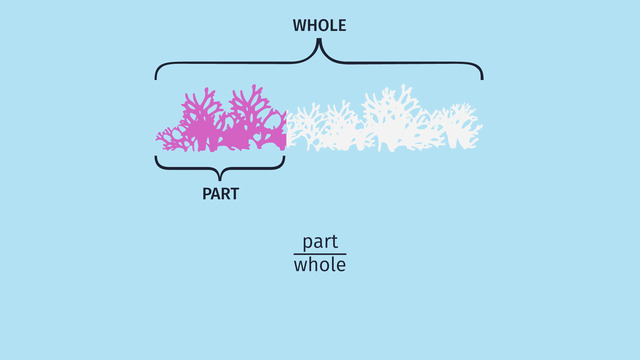# Finding One Hundred Percent Given Another PercentRating

Ø 5.0 / 1 ratings
The authorsChris S.

## Basics on the topicFinding One Hundred Percent Given Another Percent

After this lesson, you will be able to solve word problems involving finding 100% of a given quantity with and without using equations.

The lesson begins with representing a proportional relationship as a fraction and as a tape diagram. It leads you to using the factors of 100 to solve problems. It concludes with finding the original price given the percent decrease and discounted price.

Learn about percents by helping Sydney understand and adapt to the changing reef.

This video includes key concepts, notation, and vocabulary such as quantity, percent, part, whole (100% of a quantity), modified double number lines, and factors of 100.

Before watching this video, you should already be familiar with proportional relationships, percent, whole, part, factors, and multiples.

After watching this video, you will be prepared to learn...

Common Core Standard(s) in focus: 7.RP.A.1, 7.RP.A.2c A video intended for math students in the 7th grade Recommended for students who are 12 - 13 years old

### TranscriptFinding One Hundred Percent Given Another Percent

After growing up with his parents in a sheltered cove of the coral reef, Sydney is ready to move into his own place. He explores the reef far and wide, but is finding it difficult to get a place. His parents explain that a percentage of the reef has died and there is much less housing available now than there used to be. In order to understand and adapt to the changing reef, Sydney will need to find 100% given another percent. When Sydney's parents were growing up, the WHOLE reef was alive and inhabited, but today, only PART of it is still alive. We can represent this with a fraction of part to whole and also visually as a modified tape diagram. We can let the entire tape represent 100% of the reef, and today, only 40% is alive--Sydney knows that this part is 560 square miles. Sydney's parents tell him the whole reef use to be 1,400 square miles. Notice the proportional relationship that we have here. 560 over 1,400 is equal to 40 over 100. That thriving reef must have been a sight to behold! Although it's tough to find a place these days, Sydney continues his quest and stumbles across a cute apartment in a colorful neighborhood! But there's already a crowd of 15 interested sea creatures. And Sydney discovers thats only 25% of the interested applicants. So, how many creatures have actually applied to rent this apartment? Recall that percent is part out of 100. So knowing our factors of 100, can help us solve percent problems like this one. Let's list the factors by thinking of two numbers we can multiply to get 100. 1 times 100 is definitely 100. 2 times? 50 is 100. 3 times? No! Wait! Three isn't a factor of 100! But 4 and? 25 are factors of 100. So are 5 and? 20. And, of course, 10 times 10 is also 100. Going back to Sydney's problem, what information do we know? We know that 15 represents 25%, but we still need to whole 100% Luckily, our percent, 25, is one of the factors of 100. So to get 25% to be 100% we'd need to multiply by 4, seen here. And remembering that the values are proportional, we can use this factor of 4 to multiply by 15 giving us 60. Oh man, 60 sea creatures have applied to rent this apartment? That'll be tough for Sydney. Nevertheless, the price is just right--the apartment is only 425 dollars a month! That’s already 15% off the original price. Sydney is curious—what WAS the original price? Again let's create a modified tape diagram to help us and let 100% represent the original price. If 15% was discounted, what percent does the renter still have to pay? 85%. So 425 dollars is 85% of the original price. Unfortunately, 85% is not a factor of 100% but 1% is. How can we find 1% of the original price? We'd need to divide 425... by 85. How can we do this mentally? Notice that both have a common factor of 5 because they end in 5. So we can divide both evenly by 5. Let's start by breaking up 425 in terms of 400 plus 25, which are also divisible by 5. 400 divided by 5 is 80, and 25 divided by 5 is 5. Adding these values gives us 85. So if 425 divided by 5 is 85, In reverse we can see 85 times 5 is 425. Nice! Therefore, what is the greatest common factor of both 425 and 85? It is 85! We can actually divide out the common factor of 85 from both 425 dollars and 85%. This means that 5 dollars is 1%. We can find 100% by multiplying both by 100 giving us the original price of 500 dollars. Sydney is convinced that the discounted price is a great deal! Before we find out if Sydeny got the place, let’s review the tools used for finding 100% given another percent. Diagrams are useful tools which help us visualize the relationship between quantities, percents, and wholes. Given a part and its percentage, we can always find the whole. If the percentage given is a factor of 100 we can just multiply it by the other factor. If it is not a factor of 100, we can convert to a factor of 100, then also multiply to get 100. Note that 1 is a factor of 100 that you can always convert to. These tools have helped us and thanks to his persistence, Sydney got the apartment! He loves the view. But what’s this creature? This is all a little unsettling! That explains why the apartment was so cheap.